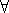## CGAL::Points_on_segment_2<Point_2>

### Definition

The class Points_on_segment_2<Point_2> is a generator for points on a segment whose endpoints are specified upon construction. The points are equally spaced

### Types

 typedef std::input_iterator_tag iterator_category; typedef Point_2 value_type; typedef std::ptrdiff_t difference_type; typedef const Point_2* pointer; typedef Point_2 reference;

### Creation

 Points_on_segment_2 g ( Point_2 p, Point_2 q, std::size_t n, std::size_t i = 0);
g is an input iterator creating points of type P equally spaced on the segment from p to q. n-i points are placed on the segment defined by p and q. Values of the index parameter i larger than 0 indicate starting points for the sequence further from p. Point p has index value 0 and q has index value n-1.
Requirement: The expressions to_double(p.x()) and to_double(p.y()) must result in the respective double representation of the coordinates of p, and similarly for q.

### Operations

 double g.range () returns the range in which the point coordinates lie, i.e.x: |x|range() andy: |y|range()
.

 Point_2 g.source () returns the source point of the segment. Point_2 g.target () returns the target point of the segment.